Previous Rule Change Main Page Resolution Decision Main Page Change List for this Rule Search Decision Number 14-02-015 Decision Number 14-02-015 Next Rule Change

Original Version
Appendix F - Part 1 - #13

 13. Longitudinal Load on Crossing Poles C and D Rule 47.5 provides that crossing structures shall withstand at all times with a safety factor of unity the unbalanced stress due to the combined pull toward the crossing of one–third of the total number of conductors supported, the pull in each such conductor being taken as the tension due to the specified loading.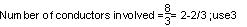Location of conductors resulting in maximum load - two on top arm and one on next arm below Bending moment: 2 x 2,605 x 48.75 = 254,000 pound–feet 1 x 2,605 x 43.25 = 112,600 pound–feet Total Moment = 366,600 pound–feet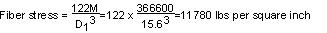The allowable value of modulus of rupture under this load is 6,000 lbs per square inch, hence poles C and D must be head guyed for longitudinal load.The head guy should be attached approximately at the normal center of load, therefore: The bending moment under full longitudinal load would be: 48.75 x 2 x 2,605 =254,000 pound–feet 43.25 x 2 x 2,605 =225,300 pound–feet 37.75 x 2 x 2,605 =196,700 pound–feet 28.33 x 2 x 570 =32,300 pound–feet Total moment =708,300 pound–feet The total longitudinal load would be: 3(2 x 2,605) =15,630 pounds 2 x 570 =1,140 pounds Total wire tensions =16,770 pounds Therefore, the center of longitudinal load is: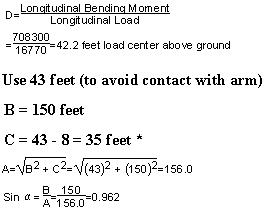* Lower end of guy assumed 8 feet above ground on Poles A and E. A guy attached at a point 43 feet above ground on pole C or D and at a point 8 feet above ground on pole B or E, respectively, would be required to withstand a load of: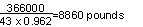In this case, a 9/16 inch common, 7/16 inch Siemens–Martin, or 3/8 inch high–strength guy strand would meet the requirements of Rule 47.5. The horizontal load transmitted to pole B or E by such a head guy would be: 8,860 x sin đ = 8,860 x 0.962 = 8,520 pounds The longitudinal moment on pole B and E would be: 8,520 x 8 = 68,160 pound–feet and the fiber stress developed in pole B or E by the tension of 9,070 pounds in the head guy would be: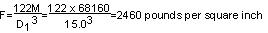Poles B and E would, therefore, be adequate to hold the contemplated guy tension with a safety factor of unity as required by Rule 47.5.

Strikeout and Underline Version
Appendix F - Part 1 - #13

 13. Longitudinal Load on Crossing Poles C and D Rule 47.547.3 provides that crossing structures shall withstand at all times with a safety factor of unity the unbalanced stress due to the combined pull toward the crossing of one–third of the total number of conductors supported, the pull in each such conductor being taken as the tension due to the specified loading.Location of conductors resulting in maximum load - two on top arm and one on next arm below Bending moment: 2 x 2,605 x 48.75 = 254,000 pound–feet 1 x 2,605 x 43.25 = 112,600 pound–feet Total Moment = 366,600 pound–feetThe allowable value of modulus of rupture under this load is 6,000 lbs per square inch, hence poles C and D must be head guyed for longitudinal load.The head guy should be attached approximately at the normal center of load, therefore: The bending moment under full longitudinal load would be: 48.75 x 2 x 2,605 =254,000 pound–feet 43.25 x 2 x 2,605 =225,300 pound–feet 37.75 x 2 x 2,605 =196,700 pound–feet 28.33 x 2 x 570 =32,300 pound–feet Total moment =708,300 pound–feet The total longitudinal load would be: 3(2 x 2,605) =15,630 pounds 2 x 570 =1,140 pounds Total wire tensions =16,770 pounds Therefore, the center of longitudinal load is:* Lower end of guy assumed 8 feet above ground on Poles A and E. A guy attached at a point 43 feet above ground on pole C or D and at a point 8 feet above ground on pole B or E, respectively, would be required to withstand a load of:In this case, a 9/16 inch common, 7/16 inch Siemens–Martin, or 3/8 inch high–strength guy strand would meet the requirements of Rule 47.547.3. The horizontal load transmitted to pole B or E by such a head guy would be: 8,860 x sin đ = 8,860 x 0.962 = 8,520 pounds The longitudinal moment on pole B and E would be: 8,520 x 8 = 68,160 pound–feet and the fiber stress developed in pole B or E by the tension of 9,070 pounds in the head guy would be:Poles B and E would, therefore, be adequate to hold the contemplated guy tension with a safety factor of unity as required by Rule 47.547.3.

Final Version
Appendix F - Part 1 - #13

 13. Longitudinal Load on Crossing Poles C and D Rule 47.3 provides that crossing structures shall withstand at all times with a safety factor of unity the unbalanced stress due to the combined pull toward the crossing of one–third of the total number of conductors supported, the pull in each such conductor being taken as the tension due to the specified loading.Location of conductors resulting in maximum load - two on top arm and one on next arm below Bending moment: 2 x 2,605 x 48.75 = 254,000 pound–feet 1 x 2,605 x 43.25 = 112,600 pound–feet Total Moment = 366,600 pound–feetThe allowable value of modulus of rupture under this load is 6,000 lbs per square inch, hence poles C and D must be head guyed for longitudinal load.The head guy should be attached approximately at the normal center of load, therefore: The bending moment under full longitudinal load would be: 48.75 x 2 x 2,605 =254,000 pound–feet 43.25 x 2 x 2,605 =225,300 pound–feet 37.75 x 2 x 2,605 =196,700 pound–feet 28.33 x 2 x 570 =32,300 pound–feet Total moment =708,300 pound–feet The total longitudinal load would be: 3(2 x 2,605) =15,630 pounds 2 x 570 =1,140 pounds Total wire tensions =16,770 pounds Therefore, the center of longitudinal load is:* Lower end of guy assumed 8 feet above ground on Poles A and E. A guy attached at a point 43 feet above ground on pole C or D and at a point 8 feet above ground on pole B or E, respectively, would be required to withstand a load of:In this case, a 9/16 inch common, 7/16 inch Siemens–Martin, or 3/8 inch high–strength guy strand would meet the requirements of Rule 47.3. The horizontal load transmitted to pole B or E by such a head guy would be: 8,860 x sin đ = 8,860 x 0.962 = 8,520 pounds The longitudinal moment on pole B and E would be: 8,520 x 8 = 68,160 pound–feet and the fiber stress developed in pole B or E by the tension of 9,070 pounds in the head guy would be:Poles B and E would, therefore, be adequate to hold the contemplated guy tension with a safety factor of unity as required by Rule 47.3.